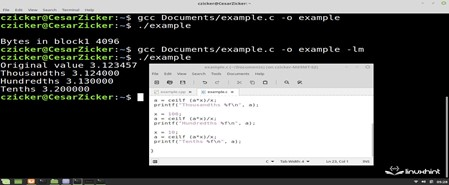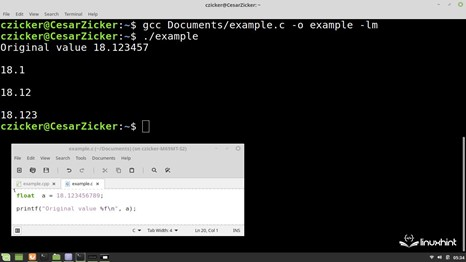| by Arround The Web | No comments

## Setting Decimal Precision in C

In practice, there are cases outside the scientific field where it’s not necessary to work with the highest precision. For example, an accounting program doesn’t need to calculate microdollars or nanodollars, let alone report them in its results.

The decimal precision with which a system operates is closely related to the number of bits it processes and also to certain standards that govern the integer and decimal lengths of floating-point variables.

Although the accuracy of a system cannot be changed, adjustments can be made to the contents of the variables through basic operations to make the use of the program that we’ll create more practical for the user.

In this Linuxhint article, you’ll learn how to adjust the decimal precision at which a program operates. We’ll look at some techniques based on simple rounding and arithmetic operations to adjust the precision of variables. We’ll also show you how to adjust the decimal precision in output functions such as printf().

## Method to Adjust the Decimal Precision of a Variable in the C Language

One method of adjusting the decimal precision of a variable is to multiply it by a factor of 10, 100, or some other multiple of 10 depending on what precision you want to achieve, and round the result with the ceilf() function. Finally, divide the result by the same factor that it was multiplied by.

Next, we see a code snippet with the formula that applies this method which, in this case, adjusts the precision of the float to hundredths:

float x;
float a = ceilf (a *x)/x;

The number of zeros that are assigned to the multiplication factor of “x” corresponds to the number of precision digits that the variable has starting from the decimal point, for example. The decimal precision of x = 100 results in a precision of 1 hundredth.

It’s important that rounding up is always done with the ceilf() function, since rounding down with floorf() can lead to inaccurate results in practice.

## How to Adjust the Decimal Precision of a Variable in C Language

In this example, we will show you how to set the decimal precision of the floating point variable “a” with an assigned decimal value of 3.123456789. To do this, we open an empty main() function and define in it the variable “a” with the value of 3.123456789 and the variable “x” as multiplication factor, all of type float.

We use the ceilf() function to round the result of multiplying “a” by “x”, and we divide the result by “x”. We store the result in the variable “a”, so that we have the original variable with its adjusted precision.

We use the printf() function to output its result to the screen in float format. The following is the code for this example where we set the precision of “a” to thousandths, hundredths and tenths.

#include <stdio.h>
#include <stdarg.h>
#include <math.h>

void main ()

{
float  a =   3.123456789;
float x = 1000;
printf("Original value %f\n", a);

a = ceilf (a*x)/x;
printf("Thousandths %f\n", a);

x = 100;
a = ceilf (a*x)/x;
printf("Hundredths %f\n", a);

x = 10;
a = ceilf (a*x)/x;
printf("Tenths %f\n", a);

}

It’s important to note that the mathematical library that is used by the rounding functions must be called with -lm when compiling from the command line. Let’s look at the correct way to do this in GCC in the following example:

~\$ gcc Path/filename.c -o output -lm

The following image shows the compilation and execution of the code. As we can see, the decimal places in all three cases after the specified digit were rounded to zero:## How to Adjust the Decimal Precision in Functions of Inputs and Outputs in C Language

The output functions support the setting of the decimal precision of the values to be displayed. In this case, the precision is set in the format field in the function arguments after the “%” symbol.

In the printf() function or one of its variants, the fit parameter is a dot followed by the number of decimal digits to specify for precision. The following snippet shows how to set the decimal precision to thousandths using the format field of printf():

printf ("\n%.2f\n", variable );

The following code declares the variable “a” of type float and assigns it the decimal value of 18.123456789. Then, it calls the printf() function three times to display its contents on the command console.

In each case, the accuracy of the value to be displayed is adjusted by setting the accuracy in the format field after the “%” symbol. In this case, the precision is set in tenths, hundredths, and thousandths, respectively.

#include <stdio.h>
#include <stdarg.h>

void main ()

{
float  a = 18.123456789;

printf("Original value %f\n", a);
printf("\n%.1f\n", a);
printf("\n%.2f\n", a);
printf("\n%.3f\n", a);

}

The following image shows the compilation and execution of this code. As you can see, the printf() function sets the precision of the float to 1, 2, and 3 decimal digits:## Conclusion

In this Linuxhint article, we taught you how to implement the two most commonly used methods in the C language to adjust the precision of floating-point variables and how to set the precision in the functions of the standard inputs and outputs. Also, we showed you the arithmetic and rounding formulas which are applied more efficiently when adjusting the precision of variables with the float-type data.

Source: linuxhint.com